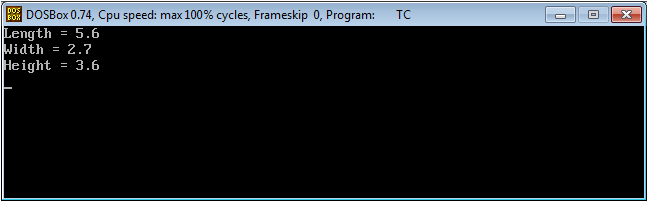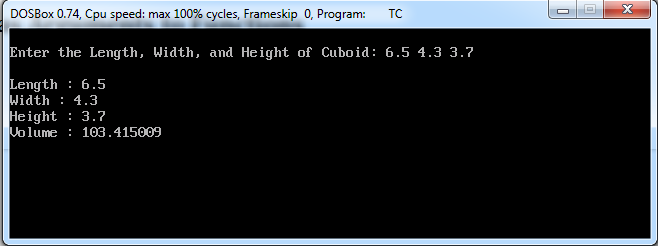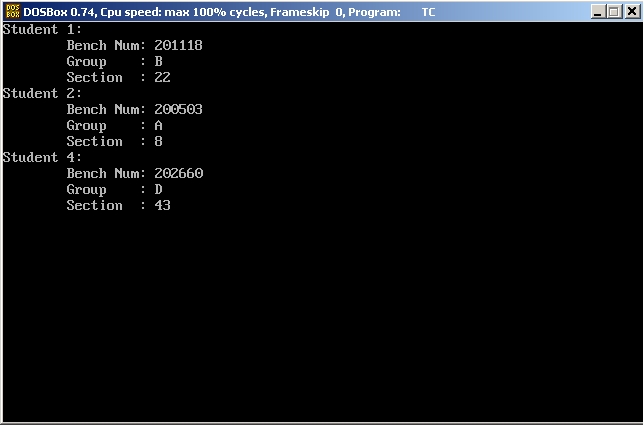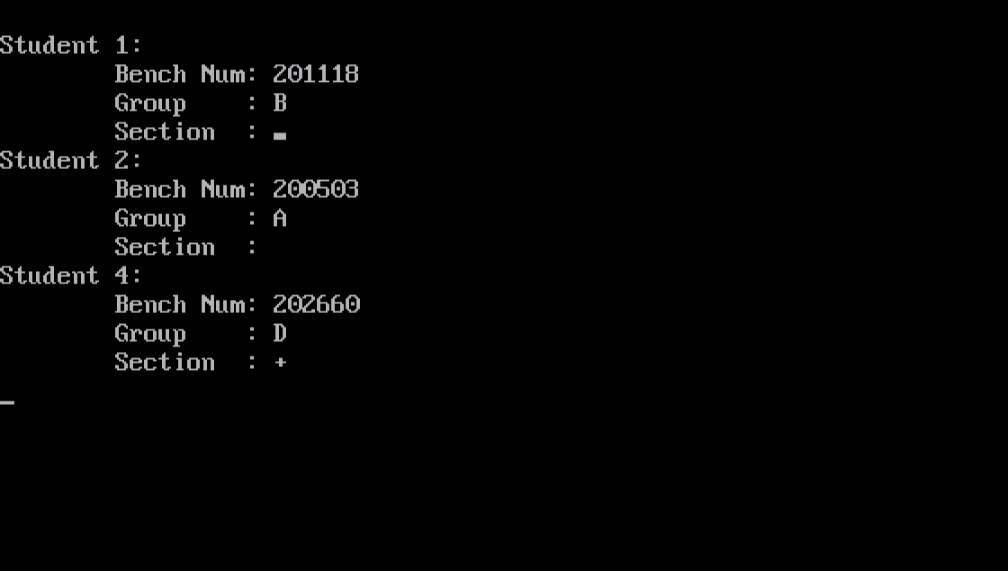# Learn about Structures in C++ Programming

0
1843So far in this series, we have worked with simple (primitive) data types. This includes types like integers (int), characters (char), and floating-point numbers (float). However, life is not that simple all the time. You may come into situations wherein you need to “logically” group (combine) a number of data items that belong to different data types together into a larger construct. Defining and using these constructs will be the subject of this article. An interesting topic to read; so, enjoy!

Structures
A Structure is (the definition from Object Oriented Programming in C++ – Robert Lafore) a collection of simple variables. As I told you above, the variables could belong to different data types. Structures can be thought of as one way to create user-defined data types.

Why Structures?
If you need to calculate area or perimeter of a circle, or the volume of a sphere, the only piece of data required is the radius of the circle (or the sphere). But, what about shapes like Cuboids?! As we know from geometry, cuboid has a length, width, and height. Despite being three different numeric variables, they are all related somehow to each other as they all represent sides of the same shape. So, it will be convenient to logically group the three variables together in a structure.

Syntax

```struct STRUCTNAME
{
MEMBER_VARIABLES_DEFINITIONS
};
```

Once defined, a structure can be treated as any other data type, so that variables could be declared to have this new structure as their type. Let’s simplify things with an example.

Example
The cuboid example will be a fine one to illustrate the idea of structures. Read with me the following program:

```#include<iostream.h>
#include<conio.h>
struct cuboid
{
float length, width, height;
};
void main()
{
clrscr();
cuboid C1;
C1.length = 5.6;
C1.width = 2.7;
C1.height = 3.6;
cout << "Length = " << C1.length << endl
<< "Width = "  << C1.width  << endl
<< "Height = " << C1.height << endl;
getch();
}
```

When executed, it will print the values of the length, width, and height:Now, to the explanation:

• The structure definition:
```struct cuboid
{
float length, width, height;
};
```

Defines a new structure named cuboid, with three member variables all of type float: length, width, and height.

• The statement
`cuboid C1;`

declares a new variable C1 of type cuboid.

• To access a member variable inside a variable of user-defined structure type, we need to use the dot operator:
`C1.length = 5.6;`

This is to assign value to a member variable. To read the value of a member variable, the same dot operator is used:

`cout << C1.length << endl;`

Structures as Arguments to Functions
Variables of type struct can be also passed as arguments to functions. Let’s modify the Cuboid example to calculate the volume.

Example
Consider this modified version of the Cuboid program:

```#include<iostream.h>
#include<conio.h>
struct cuboid
{
float length, width, height;
};
float volume(cuboid c)
{
return c.length * c.width * c.height;
}
void main()
{
clrscr();
cuboid C1;
cout << "\nEnter the Length, Width, and Height of Cuboid: ";
cin >> C1.length >> C1.width >> C1.height;
cout << "\nLength : " << C1.length << endl
<< "Width : "  << C1.width  << endl
<< "Height : " << C1.height << endl
<< "Volume : " << volume(C1)<< endl;
getch();
}
```

Output should be like the following:Easier Way to Initialize a Structure Variable
Instead of assigning values to individual member variables in a structure variable, C++ offers a more compact method. So, to assign the values 5.6 , 2.7 ,  3.6 to the member variables C1.length, C1.width, and C1.height respectively, we could use the following statement:

`cuboid C1 = { 5.6, 2.7,  3.6 };`

Surprisingly, that single statement will do the job previously done by three statements (and could be more if the structure contains more member variables).

Let’s get our hands more trained to write structures by using another example.

Example
We need to write a program that accepts and prints university students’ information. The pieces of information to work on are the student Bench Number (long), the Group (one of the characters A, B, C, or D), and the section (a number between 1 and 48). Okay, let’s do it!!

```#include <iostream.h>
#include <conio.h>
struct student
{
long BN;
char group;
char section;
};
void main()
{
clrscr();
student s1 = { 201118, 'B', 22 };
student s2 = { 200503, 'A', 8 };
student s3 = { 202660, 'D', 43 };
student s4 = s3;
cout << "Student 1:\n"
<< "\tBench Num: " << s1.BN << endl
<< "\tGroup    : " << s1.group << endl
<< "\tSection  : " << int(s1.section) << endl;
cout << "Student 2:\n"
<< "\tBench Num: " << s2.BN << endl
<< "\tGroup    : " << s2.group << endl
<< "\tSection  : " << int(s2.section) << endl;
cout << "Student 4:\n"
<< "\tBench Num: " << s4.BN << endl
<< "\tGroup    : " << s4.group << endl
<< "\tSection  : " << int(s4.section) << endl;
getch();
}
```

When executed, this program will give the following output:Now, there are few points to explain in this program:

• The statement:
`student s4 = s3;`

assigns the structure variable s3 to the structure variable s4. This has the same effect of assigning each individual member variable in s3 to the corresponding one in s4

• The group member variable was declared as char (character); that seems normal. However, the variable section was also declared as char, while it is expected to store a value between 1 and 48. How?!

The answer is easy: the char type is actually a numeric data type. It can store integer values between -128 and 127. To avoid wasting memory segments, why store an integer value that will never exceed 48, or 60, or 80, or even 120 in an integer variable (that occupies 4 bytes in memory)?!!

• The expression:
`int(s1.section)`

is called casting. Casting means a temporary (dynamic) conversion of one data type to another. For the purpose of printing 22 as 22, not as its ASCII equivalent, the section variable that was defined as character is casted (converted) dynamically on printing to its numeric value. To understand the idea, consider printing s1.section without casting:

`cout << s1.section;`Have you noticed the difference?! Great!!

Summary